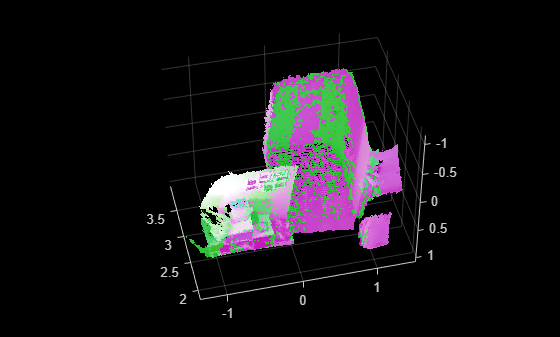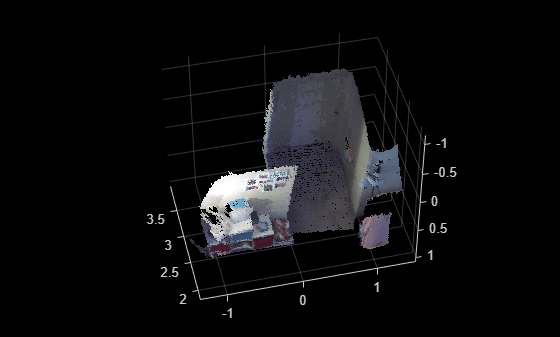# pcregisterfgr

Register two point clouds using FGR algorithm

## Syntax

``tform = pcregisterfgr(moving,fixed,gridSize)``
``[tform,rmse] = pcregisterfgr(moving,fixed,gridSize)``
``[___] = pcregisterfgr(___,MaxIterations=numIterations)``

## Description

example

````tform = pcregisterfgr(moving,fixed,gridSize)` returns a rigid transformation that registers a moving point cloud to a fixed point cloud. The function registers points using a fast global registration (FGR) algorithm based on FPFH features.```
````[tform,rmse] = pcregisterfgr(moving,fixed,gridSize)` additionally returns the root mean square error of the Euclidean distance between the inlier aligned points.```
````[___] = pcregisterfgr(___,MaxIterations=numIterations)` specifies the maximum number of iterations for the FGR algorithm, in addition to any combination of arguments from previous syntaxes.```

## Examples

collapse all

Load point cloud data into the workspace.

```ld = load("livingRoom.mat"); fixed = ld.livingRoomData{1}; moving = ld.livingRoomData{2};```

To improve the efficiency and the accuracy of the FGR registration algorithm, downsample the point clouds. Display the point clouds.

```fixedDownsampled = pcdownsample(fixed,gridAverage=0.05); movingDownsampled = pcdownsample(moving,gridAverage=0.05); pcshowpair(moving,fixed,VerticalAxis="Y",VerticalAxisDir="Down") ```Perform registration using the FGR algorithm.

```gridSize = 0.1; [tform,rmse] = pcregisterfgr(movingDownsampled,fixedDownsampled, ... gridSize,MaxIterations=100);```

Visualize the alignment.

```movingRegistered = pctransform(moving,tform); pcshowpair(movingRegistered,fixed,VerticalAxis="Y",VerticalAxisDir="Down");```Merge the point clouds and visualize the merged result.

```mergeSize = 0.01; merged = pcmerge(movingRegistered,fixed,mergeSize); figure(Name = "merged point cloud after Fast Global registration"); pcshow(merged,VerticalAxis="Y",VerticalAxisDir ="Down");```## Input Arguments

collapse all

Moving point cloud, specified as a `pointCloud` object.

Fixed point cloud, specified as a `pointCloud` object.

Grid size to search for correspondence between the point clouds, specified as a positive scalar.

Data Types: `single` | `double` | `int8` | `int16` | `int32` | `int64` | `uint8` | `uint16` | `uint32` | `uint64`

Maximum number of iterations before the FGR algorithm stops, specified as a positive integer.

Data Types: `single` | `double` | `int8` | `int16` | `int32` | `int64` | `uint8` | `uint16` | `uint32` | `uint64`

## Output Arguments

collapse all

Rigid transformation, returned as a `rigidtform3d` object. The rigid transformation registers a moving point cloud to a fixed point cloud. The `rigidtform3d` object describes the rigid 3-D transform. The FGR algorithm estimates the rigid transformation between the moving and fixed point clouds.

Root mean square error, returned as a positive scalar that represents the Euclidean distance between the inlier aligned points. A lower error values indicates a better registration.

## Algorithms

This figure shows the workflow of point cloud registration using the FGR algorithm.## Version History

Introduced in R2022b